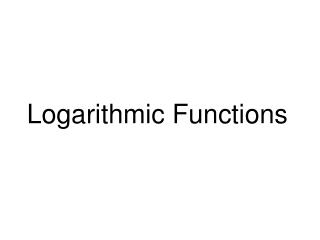DownloadDownload PresentationLogarithmic Functions

# Logarithmic Functions

Télécharger la présentation## Logarithmic Functions

- - - - - - - - - - - - - - - - - - - - - - - - - - - E N D - - - - - - - - - - - - - - - - - - - - - - - - - - -
##### Presentation Transcript

1. Logarithmic Functions

2. Definition of a Logarithmic Function For x > 0 and b > 0, b = 1, y = logb x is equivalent to by = x. The function f (x) = logb x is the logarithmic function with base b.

3. Exponent Exponent Base Base Location of Base and Exponent in Exponential and Logarithmic Forms Logarithmic form: y = logb x Exponential Form: by = x.

4. a. 2 = log5x means 52 = x. b. 3 = logb 64 means b3 = 64. Logarithms are exponents. Logarithms are exponents. Logarithms are exponents. Logarithms are exponents. Text Example Write each equation in its equivalent exponential form. a. 2 = log5x b. 3 = logb 64 c. log3 7 = y Solution With the fact that y = logbx means by = x, c. log3 7 = y or y = log3 7 means 3y = 7.

5. Logarithmic Expression Question Needed for Evaluation Logarithmic Expression Evaluated a. log2 16 2 to what power is 16? log2 16 = 4 because 24 = 16. b. log3 9 3 to what power is 9? log3 9 = 2 because 32 = 9. c. log25 5 25 to what power is 5? log25 5 = 1/2 because 251/2 = 5. Text Example Evaluate a. log2 16 b. log3 9 c. log25 5 Solution

6. Basic Logarithmic Properties Involving One • Logbb = 1 because 1 is the exponent to which b must be raised to obtain b. (b1 = b). • Logb 1 = 0 because 0 is the exponent to which b must be raised to obtain 1. (b0 = 1).

7. For x > 0 and b  1, logb bx = x The logarithm with base b of b raised to a power equals that power. b logb x = x b raised to the logarithm with base b of a number equals that number. Inverse Properties of Logarithms

8. General PropertiesCommon Logarithms 1. logb 1 = 0 1. log 1 = 0 2. logbb = 1 2. log 10 = 1 3. logbbx = 0 3. log 10x = x 4. b logb x = x 4. 10 log x = x Properties of Common Logarithms

9. log 4 4 = 1 log 8 1 = 0 3 log 3 6 = 6 log 5 5 3 = 3 2 log 2 7 = 7 Examples of Logarithmic Properties

10. General PropertiesNatural Logarithms 1. logb 1 = 0 1. ln 1 = 0 2. logbb = 1 2. ln e = 1 3. logbbx = 0 3. ln ex = x 4. b logb x = x 4. eln x = x Properties of Natural Logarithms

11. e log e 6 = e ln 6 = 6 log e e 3 = 3 Examples of Natural Logarithmic Properties

12. Problems Use the inverse properties to simplify:

13. 6 6 5 5 4 4 3 3 2 2 -2 -1 2 3 4 5 6 -2 -1 2 3 4 5 6 -1 -1 f (x) = logbx 0<b<1 f (x) = logbx b>1 -2 -2 Characteristics of the Graphs of Logarithmic Functions of the Form f(x) = logbx • The x-intercept is 1. There is no y-intercept. • The y-axis is a vertical asymptote. (x = 0) • If 0 < b < 1, the function is decreasing. If b > 1, the function is increasing. • The graph is smooth and continuous. It has no sharp corners or edges.

14. x -2 -1 0 1 2 3 x 1/4 1/2 1 2 4 8 f (x) = 2x 1/4 1/2 1 2 4 8 g(x) = log2 x -2 -1 0 1 2 3 Text Example Graph f (x) = 2x and g(x) = log2x in the same rectangular coordinate system. Solution We first set up a table of coordinates for f (x) = 2x. Reversing these coordinates gives the coordinates for the inverse function, g(x) = log2x. Reverse coordinates.

15. y = x f (x) = 2x 6 5 4 3 f (x) = log2x 2 -2 -1 2 3 4 5 6 -1 -2 Text Example Graph f (x) = 2x and g(x) = log2x in the same rectangular coordinate system. Solution We now sketch the basic exponential graph. The graph of the inverse (logarithmic) can also be drawn by reflecting the graph of f (x) = 2x over the line y = x.

16. Examples Graph using transformations.

17. Domain of Logarithmic Functions Because the logarithmic function is the inverse of the exponential function, its domain and range are the reversed. The domain is { x | x > 0 } and the range will be all real numbers. For variations of the basic graph, say the domain will consist of all x for which x + c > 0. Find the domain of the following: 1. 2. 3.

18. Sample Problems Find the domain of